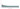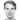# Calcul d'une levée de fonds avec des obligations convertibles

Les levées de fonds peuvent devenir délicates à calculer lorsque des obligations convertibles avec plafonnement entrent en jeu. Voyons donc comment cela fonctionne.

This page is only available in English

Investment rounds can get tricky to calculate when convertible rounds with cap and discount come into play. So let’s have a look at how that works. First, what’s a convertible? It’s, in short, an investment in a company which is done outside of a financing round and then converted (or sometimes paid back) at the next round. This is useful for a number of cases, most importantly for seed-rounds when the valuation is still open. It also reduces the administrative effort needed and allows for quick capital injections under well-defined terms.

The calculation of the number of shares which each of the investors gets can be confusing, especially for founders with less experience and when additional parameters like valuation cap, interest, and discount come into play. There are some slight differences in how this is done by different people and we implemented the most usual cases. The following explains how the numbers in Ledgy are calculated.

The first step is to calculate the $\text{PricePerShare}$. In the most simple case, without a $\text{Cap}$ or $\text{Discount}$, the share price is the pre-money $\text{Valuation}$ divided by the total number of shares ($\text{PreMoneyShares}$). This includes both shares currently issued by the company, as well as option pools and options, as they will convert to shares later (fully-diluted). The resulting price is often rounded to two decimals.

$\text{PricePerShare} = \text{Valuation} / \text{PreMoneyShares}$

A cap puts a maximum on the valuation used. This protects the investor from getting very little shares in case the company valuation goes through the roof. A discount also protects the investor, but in the other direction: If the company doesn’t perform as well as anticipated, the person who gave the convertible loan gets more shares than a new investor. For a range of valuations, this would look like in the chart below.Example for the stake which an investor will get for a range of company valuations

So mathematically speaking, a cap and a discount do the following: For a given valuation the smaller value of the discounted valuation and the cap is taken instead of the valuation:

$\text{PricePerShare} = \frac{\min{\Big[ \text{Valuation} * (1 - \text{Discount}),\;\text{Cap} \Big]}}{\text{PreMoneyShares}}$

If the investment has an interest, the time difference between the issue date and the conversion date and a daily interest rate of $\text{Interest}/365$ is used to calculate the increase of the investment (sometimes 360 days are used instead, which can be defined in Ledgy):

$\text{Investment’} = \text{Investment} * \left(1 + \frac{\text{Interest} * \text{Days}}{365} \right)$

The number of shares is simply calculated from the investment and the share price as

$\text{ConvertibleShares} = \frac{\text{Investment’}}{\text{PricePerShare}}$

The number of shares is then rounded to the closest integer, or rounded down (can be defined in Ledgy’s round modeling), as there’s no such thing as a fraction of a company share. But does the investor just get a wrong number of shares in this situation? Usually not. The investment amount is reverse-calculated from the rounded number of shares ($\text{ConvertibleShares}$) like

$\text{Investment’’} = \text{ConvertibleShares} * \text{PricePerShare}$

This is then the real amount of money the investor will transfer to the company’s bank account. Additionally, when you're modeling or calculating a round or exit chart you should pay attention to anti-dilution provisions and know all that is included when you're fully diluted.

04 Dec 2017